Examples

Chapter 6 Class 12 Application of Derivatives
Serial order wise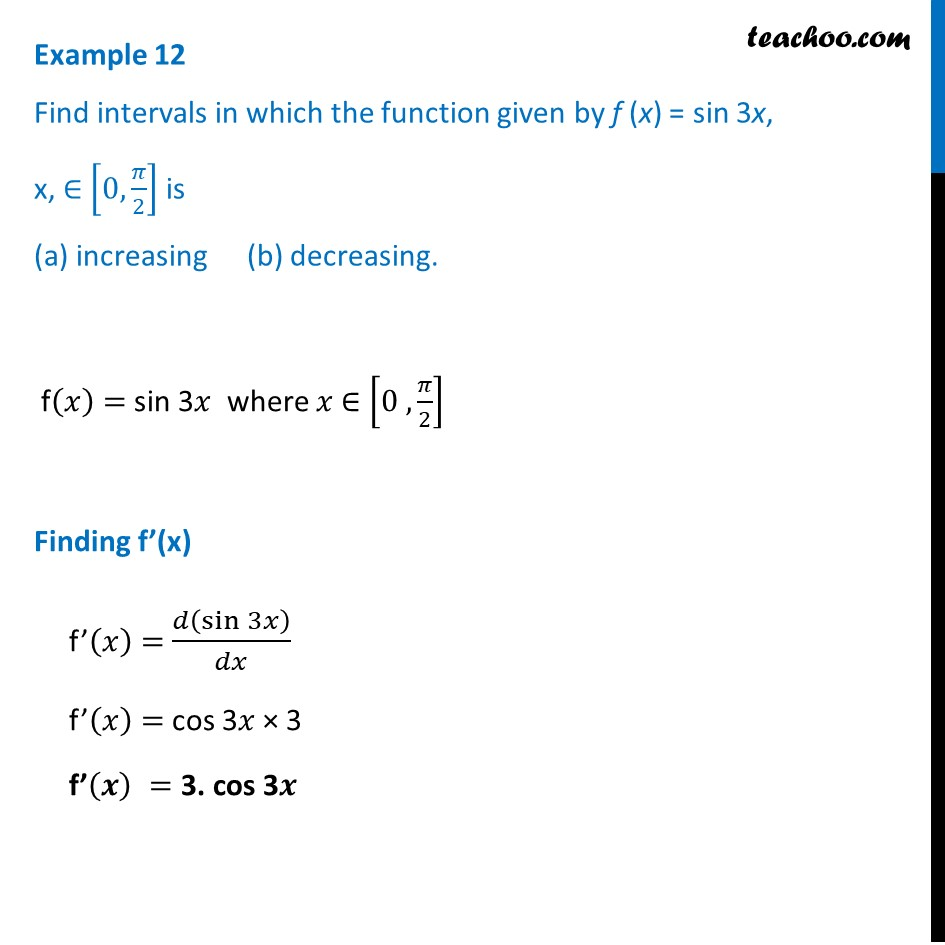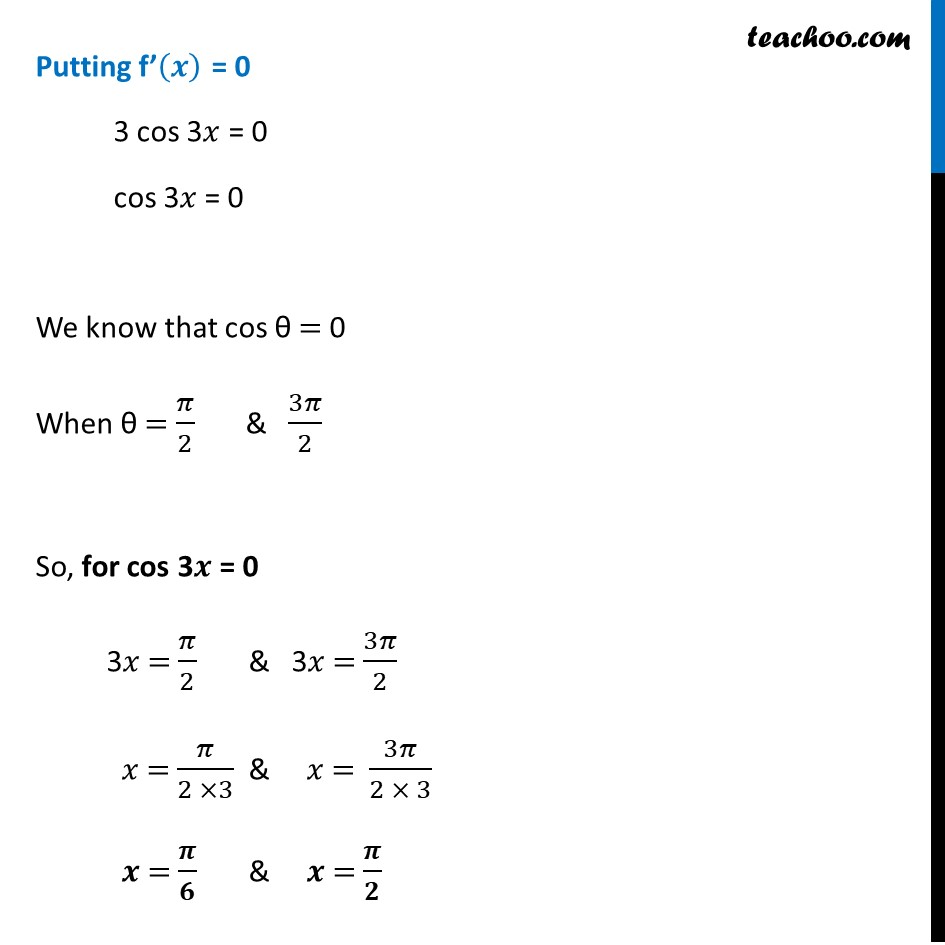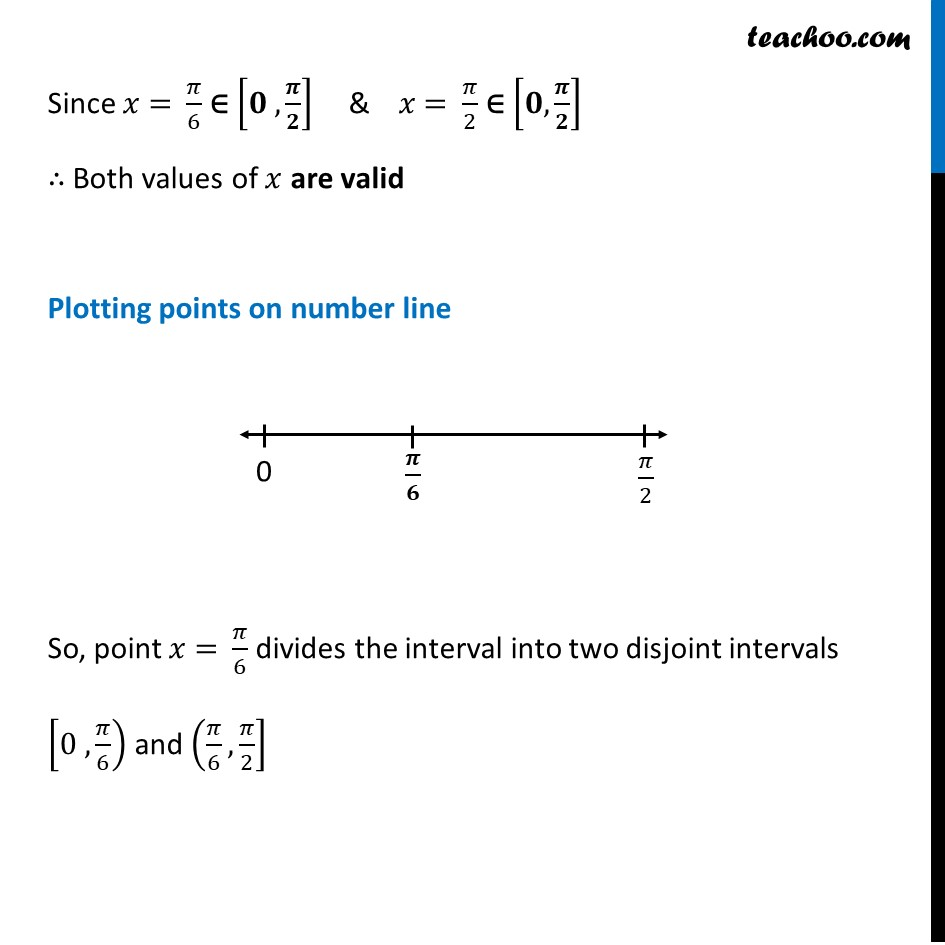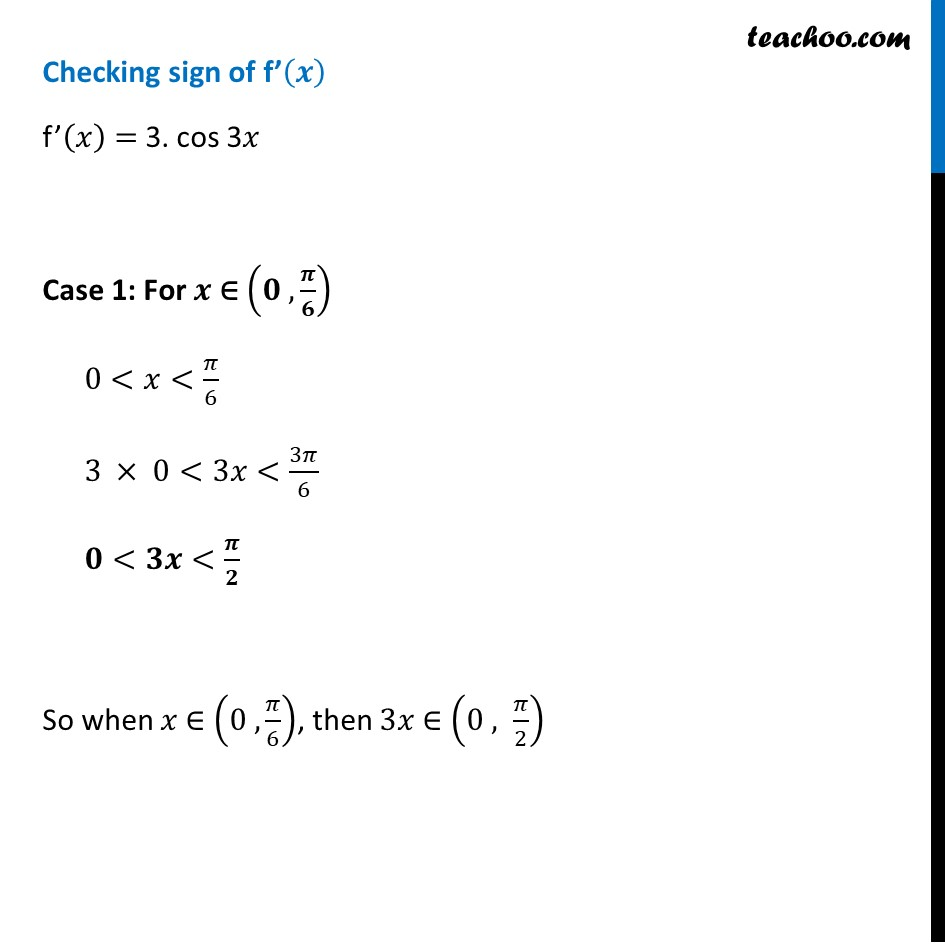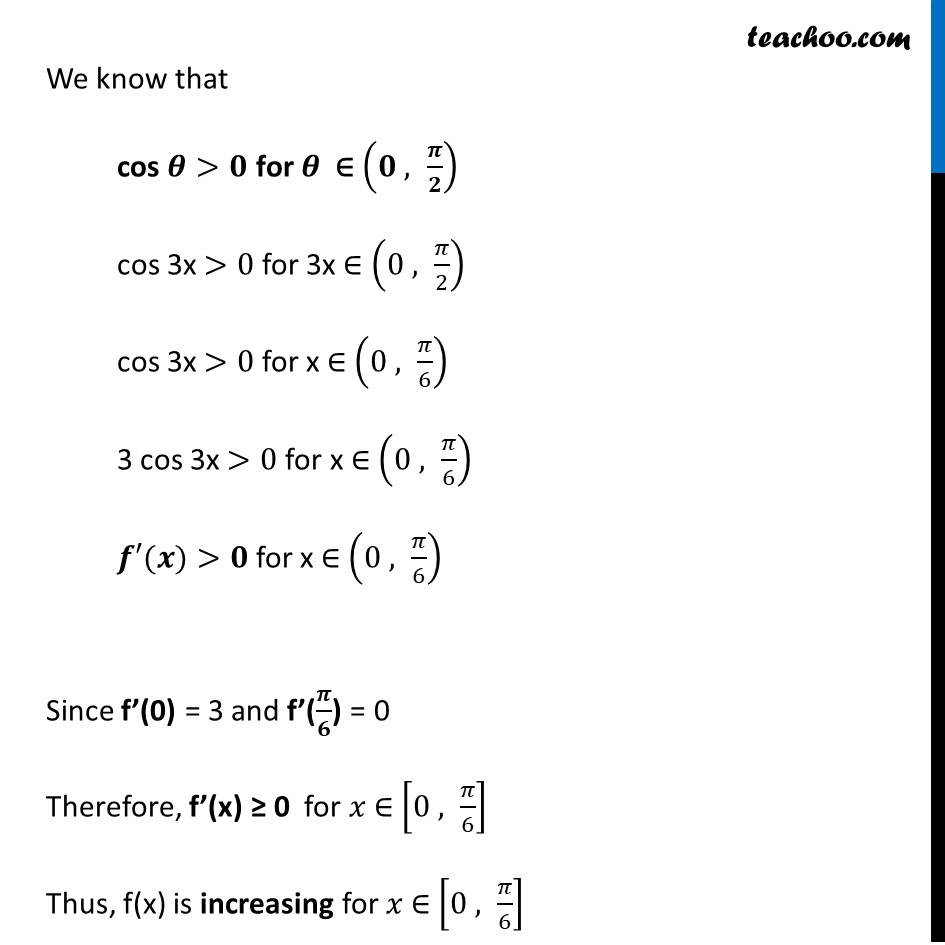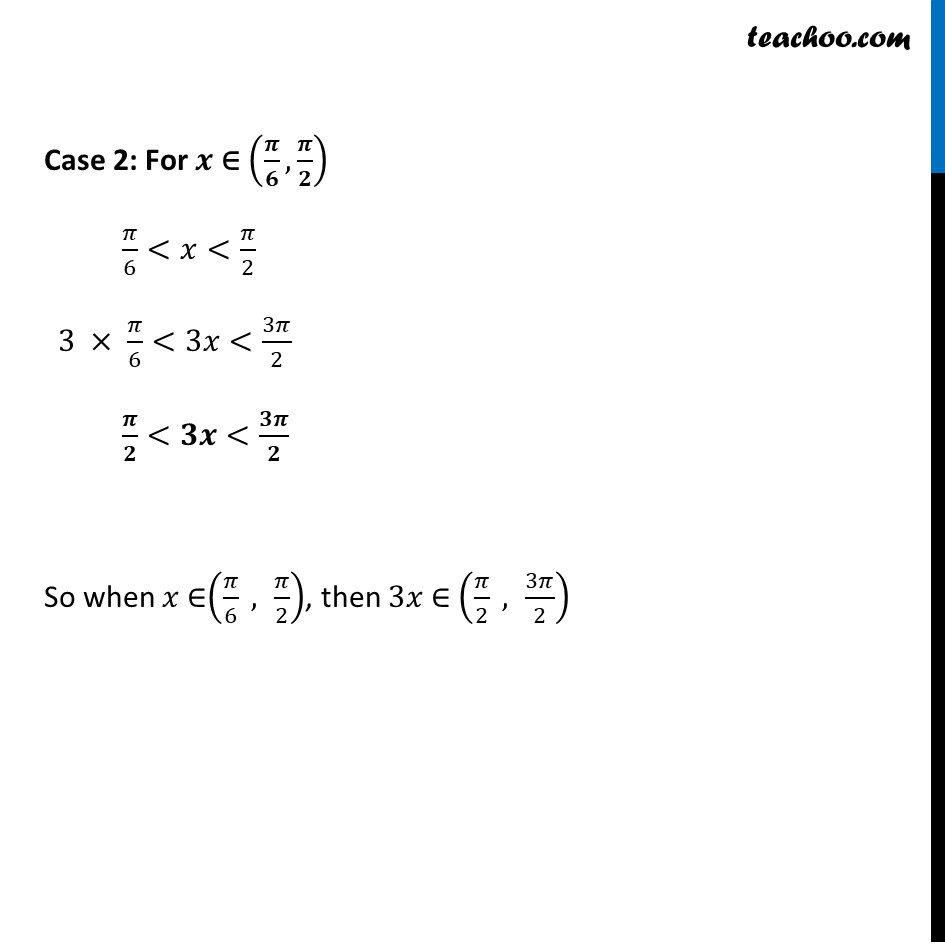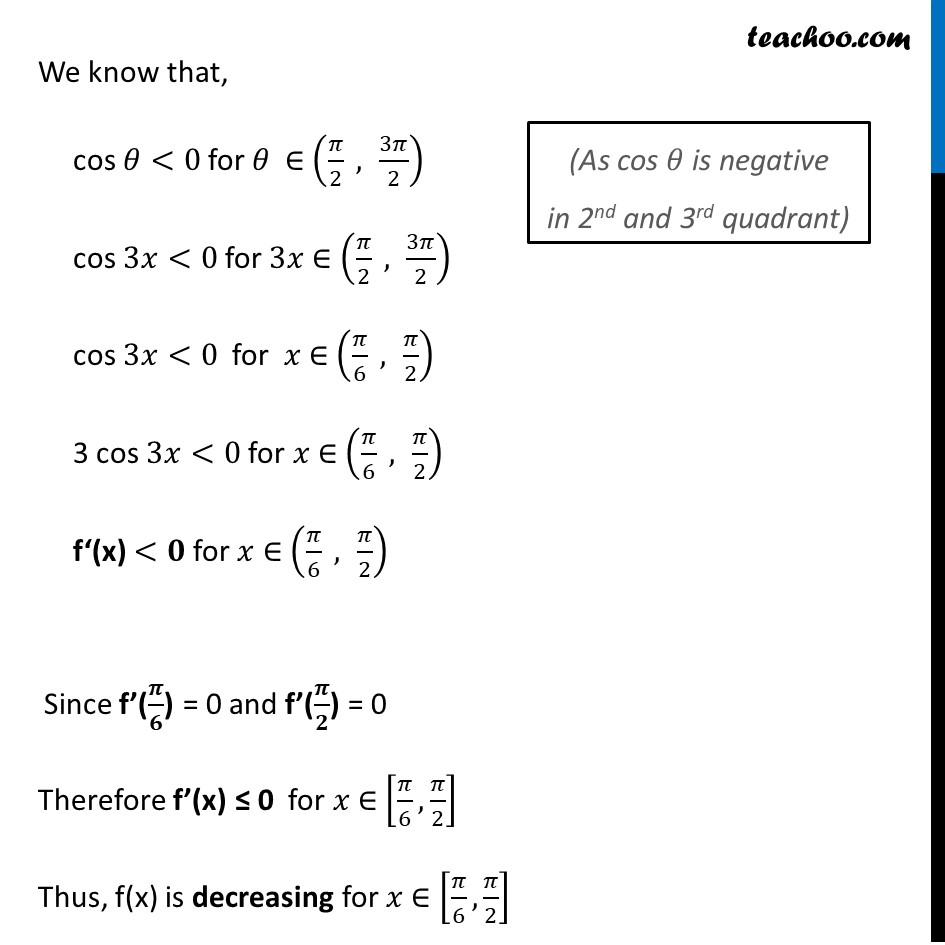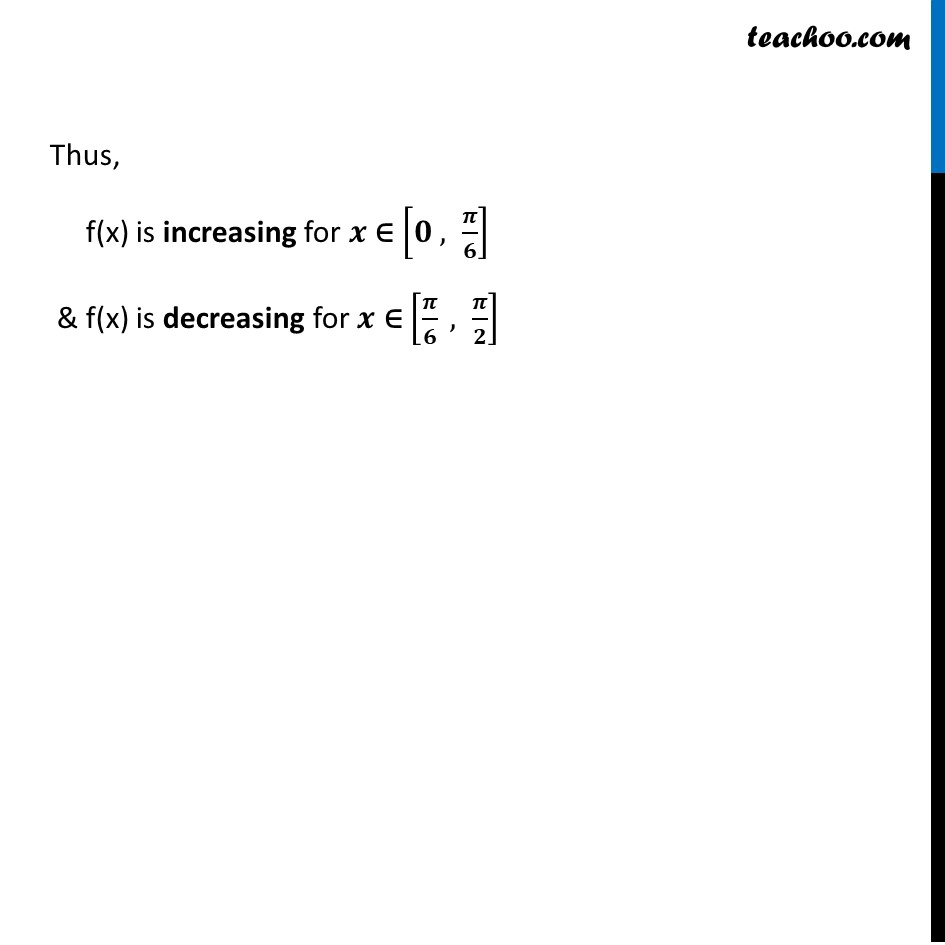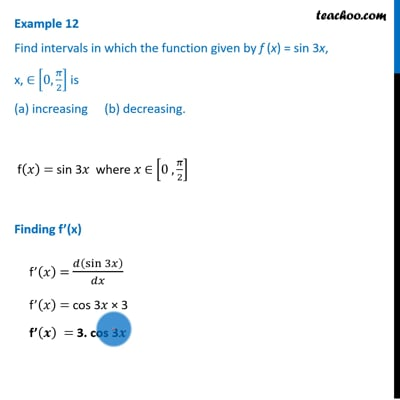This video is only available for Teachoo black users

Maths Crash Course - Live lectures + all videos + Real time Doubt solving!

### Transcript

Example 12 Find intervals in which the function given by f (x) = sin 3x, x, ∈ [0, 𝜋/2] is (a) increasing (b) decreasing. f(𝑥) = sin 3𝑥 where 𝑥 ∈ [0 ,𝜋/2] Finding f’(x) f’(𝑥) = 𝑑(sin⁡3𝑥 )/𝑑𝑥 f’(𝑥) = cos 3𝑥 × 3 f’(𝒙) = 3. cos 3𝒙 Putting f’(𝒙) = 0 3 cos 3𝑥 = 0 cos 3𝑥 = 0 We know that cos θ = 0 When θ = 𝜋/2 & 3𝜋/2 So, for cos 3𝒙 = 0 3𝑥 = 𝜋/2 & 3𝑥 = 3𝜋/2 𝑥 = 𝜋/(2 ×3) & 𝑥 = 3𝜋/(2 × 3) 𝒙 = 𝝅/𝟔 & 𝒙 = 𝝅/𝟐 Since 𝑥 = 𝜋/6 ∈ [𝟎 ,𝝅/𝟐] & 𝑥 = 𝜋/2 ∈ [𝟎,𝝅/𝟐] ∴ Both values of 𝑥 are valid Plotting points on number line So, point 𝑥 = 𝜋/6 divides the interval into two disjoint intervals [0 ,𝜋/6) and (𝜋/6, 𝜋/2] Checking sign of f’(𝒙) f’(𝑥) = 3. cos 3𝑥 Case 1: For 𝒙 ∈ (𝟎 ,𝝅/𝟔) 0<𝑥<𝜋/6 3 × 0<3𝑥<3𝜋/6 𝟎<𝟑𝒙<𝝅/𝟐 So when 𝑥 ∈ (0 ,𝜋/6), then 3𝑥 ∈ (0 , 𝜋/2) We know that cos 𝜽>𝟎 for 𝜽 ∈ (𝟎 , 𝝅/𝟐) cos 3x >0 for 3x ∈ (0 , 𝜋/2) cos 3x >0 for x ∈ (0 , 𝜋/6) 3 cos 3x >0 for x ∈ (0 , 𝜋/6) 𝒇′(𝒙)>𝟎 for x ∈ (0 , 𝜋/6) Since f’(0) = 3 and f’(𝝅/𝟔) = 0 Therefore, f’(x) ≥ 0 for 𝑥 ∈ [0 , 𝜋/6] Thus, f(x) is increasing for 𝑥 ∈ [0 , 𝜋/6] Case 2: For 𝒙 ∈ (𝝅/𝟔, 𝝅/𝟐) 𝜋/6<𝑥<𝜋/2 3 × 𝜋/6<3𝑥<3𝜋/2 𝝅/𝟐<𝟑𝒙<𝟑𝝅/𝟐 So when 𝑥 ∈(𝜋/6 , 𝜋/2), then 3𝑥 ∈ (𝜋/2 , 3𝜋/2) We know that, cos 𝜃<0 for 𝜃 ∈ (𝜋/2 , 3𝜋/2) cos 3𝑥<0 for 3𝑥 ∈ (𝜋/2 , 3𝜋/2) cos 3𝑥<0 for 𝑥 ∈ (𝜋/6 , 𝜋/2) 3 cos 3𝑥<0 for 𝑥 ∈ (𝜋/6 , 𝜋/2) f‘(x) <𝟎 for 𝑥 ∈ (𝜋/6 , 𝜋/2) Since f’(𝝅/𝟔) = 0 and f’(𝝅/𝟐) = 0 Therefore f’(x) ≤ 0 for 𝑥 ∈ [𝜋/6,𝜋/2] Thus, f(x) is decreasing for 𝑥 ∈ [𝜋/6,𝜋/2] (As cos 𝜃 is negative in 2nd and 3rd quadrant) Thus, f(x) is increasing for 𝒙 ∈ [𝟎 , 𝝅/𝟔] & f(x) is decreasing for 𝒙 ∈ [𝝅/𝟔 , 𝝅/𝟐]